# What does 'critical' mean? Experimental observations via stochastic sampling versus intrinsic system properties.

Claudius Gros, Dimitrije Markovic

Institut für theoretische Physik
Goethe-Universität Frankfurt a.M.

# Overview

### generating powerlaws: established (?) routes

• sandpiles - conserved dynamics with forced large events
• preferential attachement - rich gets richer
• continuously active networks (brain) - critical branching
• constrained information optimization +
logarithmic discounting (Weber-Fechner)

### attractors in critical systems

• large number of 'small' attractors may dominate intrinsic state
• small number of 'big' attractors may dominate
observations (sampling) and biological properties
• NK / vertex routing models

# infinite time-scale separation in sandpiles×[C. Gros, Complex and Adaptive Dynamical Systems, Springer 2008/10/13/15]

• fat tails forced (sand can be lost only at the boundaries)
• conserved dynamics
$\ \color{darkRed}\Longrightarrow\$ mapping to critical branching $(p=1/2)$ in infinite dimesion
$\ \color{darkRed}\Longrightarrow\$ powerlaws×# rich gets richer

Internet 2011: in-degree distributionlarge-scale crawl, 32 Million most important sites, 2011
×[Gros, Kaczor, Markovic, EPJB 2012,
Neuropsychological constraints to human data production on a global scale]

- data availabe for further analysis -

### generating powerlaws via preferential attachment

[Barabasi, Albert, ...]
• probability of external attachment: $\ \color{darkGreen} r$
• probability of internal attachment: $\ \color{darkGreen}{1-r}$
$$p_k\ \sim\ \frac{1}{k^\gamma},\qquad \gamma = 1+\frac{1}{1-r/2}$$ $\ \color{darkRed}\Longrightarrow\$ mostly internal growth
• distribution of citations
• ...

# continuously active networks

[Markovic, Gros, Powerlaws and Self-Organized Criticality in Theory and Nature, Physics Reports 2014]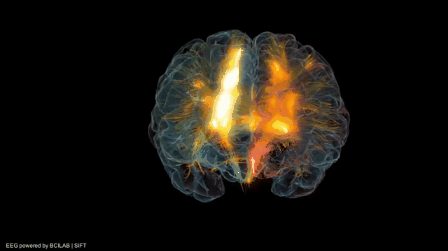[Gazzaley & Rosedale]×

## absorbing phase transition: external drivings couple to order parameter×

## external fields coupling to order parameter destroy the phase transition• brain is autonomous & continuously active
• homeostatically regulated
• time scale $\,\gg\,$ avalanche duration
• interacting network of responsive neurons
• $\color{darkRed}\Longrightarrow\$ equivalent to critical
$\phantom{\Longrightarrow}\ \$ branching $\ (p=1/2)$
• spontaneously active neural centers
• inputs (coupling to order parameter)
sensory & from other brain areas

## two viewpoints

 $\ \color{darkRed}\Longrightarrow\$ slightly subcritical $\ (p<1/2)$ :: save distance from chaotic region? $\ \color{darkRed}\Longrightarrow\$ critical point destroyed :: close to Widom line? :: (line of maximal response)

# humans maximizing Shannon information

### maximal entropy distributions

with constraint $\displaystyle\quad \langle x\rangle=\mathrm{const.} \qquad\quad\color{darkBlue}{p(x)\ \propto\ \mathrm{e}^{-\lambda x} }$
• e.g. Boltzmann factor $\ \ \mathrm{e}^{-\beta H}$
• $\beta=1/(k_B T)$:   Lagrange multiplier

### Weber-Fechner law

$$\begin{array}{llcll} \color{darkGreen}{\mathrm{music:}} & \mathrm{tone\ pitch} &\propto& \log(\mathrm{frequency}) & \color{darkGreen}{\mathrm{(octave)}} \\ \color{darkGreen}{\mathrm{photometry:}} & \mathrm{brightness} &\propto& \log(\mathrm{intensity}) & \color{darkGreen}{\mathrm{(lumen)}} \\ \color{darkGreen}{\mathrm{acoustics:}} & \mathrm{sound\ level} &\propto& \log(\mathrm{intensity}) & \color{darkGreen}{\mathrm{(decibel)}} \\ \end{array}$$
• humans discount most sensory inputs
logarithmically
• sound/light intensities
• number of objects
• time, ...
$$\color{darkBlue}{x\ \to\ \log(x)} \qquad \color{darkRed}{\Rightarrow} \qquad \color{darkBlue}{p(x)\ \to\ \mathrm{e}^{-\lambda \log(x)} \ \propto\ \frac{1}{x^\gamma} }$$ $\ \color{darkRed}\Longrightarrow\$ scale invariant distributions

# publicly available data files in the internet[Gros, Kaczor, Markovic, EPJB 2012]

• 2011: massive crawl
• 32 million hosts
• 600 million data files
• Mime type classification
 image/ : 58.0% application/ : 33.2% text/ : 5.8% audio/ : 2.9% video/ : 0.7%
• compression
• image/gif: lossless
• image/jpeg: lossy
kink at 4M: amateur/professional?

# distribution of 2-dimensional data files[Gros, Kaczor, Markovic, EPJB 2012]

 $\ \color{darkGreen}\Longrightarrow\$ log-normal distributions

• file size (modulo compression)
• video/
time $\times$ (bytes per frame)
• audio/
time $\times$ (frequency resolution)

# maximal entropy distributions for 2D data

• $xy$ - physical size of file
• $x^2$, $y^2$ - marginal variances
• maximal entropy distribution
$$\color{darkBlue}{ p(x,y)\ \ \propto\ \ \mathrm{e}^{-\,\lambda_1\, xy\, -\,\lambda_2\, x^2\,-\,\lambda_3\, y^2} \ \ =\ \ \mathrm{e}^{-\,\lambda[\alpha\color{darkGreen}{(x+y)}\,+\, \beta\color{darkGreen}{(x-y)}]^2} }$$ logarithmic discounting $$\quad \quad \color{darkGreen}{ x+y \ \ \to\ \ \log(xy) \qquad\qquad x-y \ \ \to\ \ \log(x/y) }$$×[Gros, Kaczor, Markovic, EPJB 2012]
• only total size $\ \color{darkGreen}{xy}\$ is externaly observable

$\color{darkOrange}\Longrightarrow\$ average over $\ \color{darkGreen}{\log(x/y)}\$
$\color{darkOrange}\Longrightarrow\$ log-normal distribution
$$\color{darkBlue}{ p(x,y)\ \ \propto\ \ \mathrm{e}^{-\,\lambda''\, \log^2(xy)\, -\,\lambda'\, \log(xy)} }$$

# rank count vs. count probability

[Zipf '49, Cancho & Sole 03]$\qquad\quad$

### 40.000 most frequent (english) words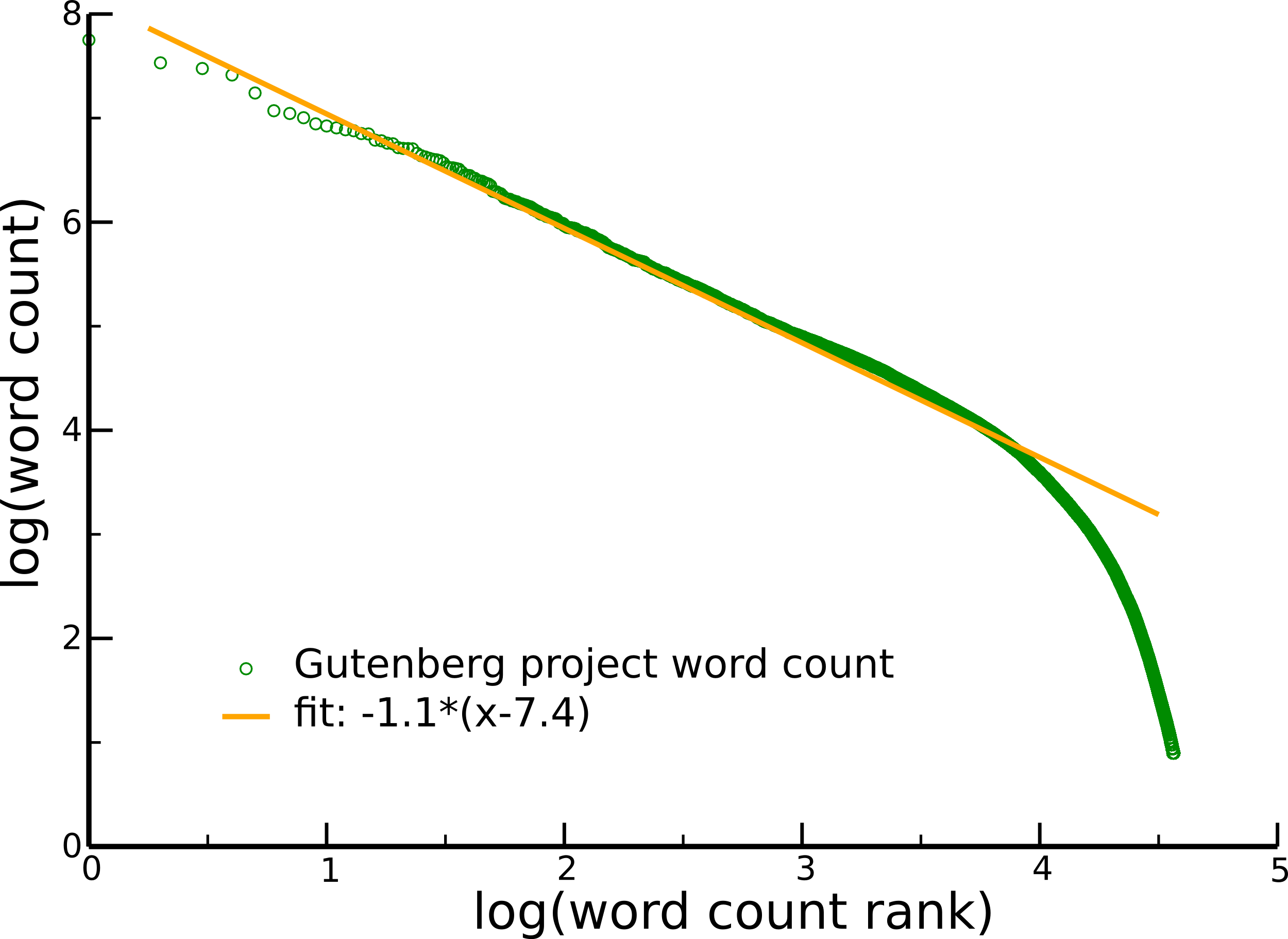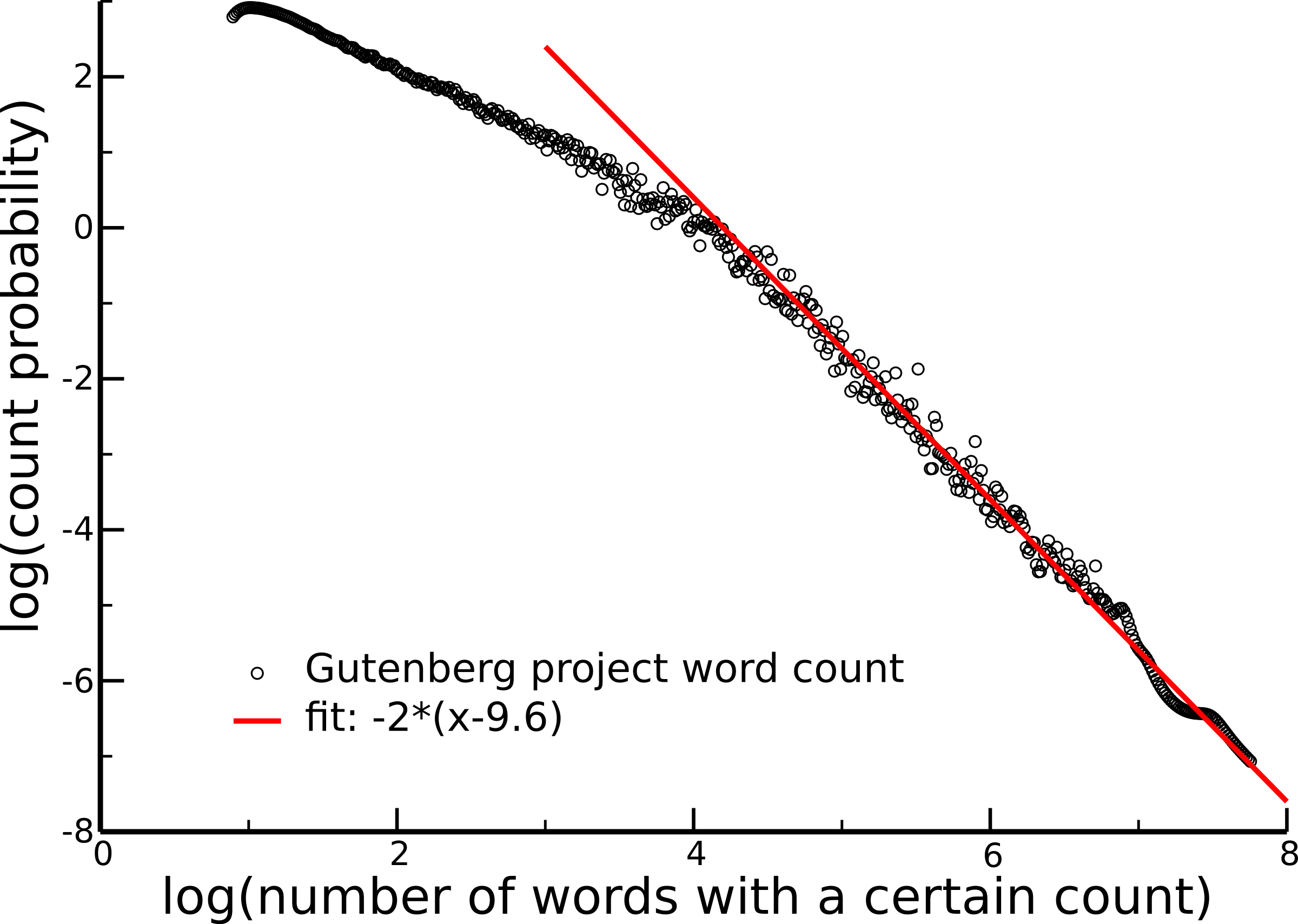$$\color{orange}{\left(\frac{1}{R}\right)^\gamma}$$ $$\color{red}{\left(\frac{1}{C}\right)^{1+1/\gamma}}$$

# wrap up - generating powerlaws

### some stablished routes

• sandpiles / continuously autonomous active system
:: mapping to critical branching
• preferential attachement - rich gets richer
• constrained information optimization by humans
:: human neurophysiology (Weber-Fechner)
• mechanisms generating general fat tails

### ... next: observing powerlaws

• the role of small attractors

# NK / Kauffman networks[Luque & Sole, ‘00]

$$\begin{array}{rcl} N & : & \mathrm{number\ of\ sites} \\ K & : & \mathrm{number\ of\ controlling\ elements} \\ \Sigma &= & (\sigma_1,\dots,\sigma_N), \qquad\quad \sigma_i=\pm1 \end{array}$$×
 N=7 K=3state perturbed state

converge, if within the same basin of attraction

### number of (cyclic) attrators

$$\begin{array}{rcl} \color{darkOrange}{\mathrm{Kauffman\ '69:}} &\sim& \sqrt{N} \\ &\Rightarrow& \color{darkGreen}{\mathrm{cell\ differentiation}}\\[1.0ex] \color{darkOrange}{\mathrm{Samuelsson\ \&\ Troein\ '03:}} &>& O(N^p) \ \ \mbox{(any p)} \end{array}$$

# vertex routing models

### transmission vs. routing

 transmission routing vertex $\ \color{darkOrange}{\Rightarrow}\$ vertex link (incomming) $\ \color{darkOrange}{\Rightarrow}\$ link (outgoing) dynamical variables: vertices dynamical variables: links $\qquad\qquad$[Markovic & Gros, New Journal of Physics 09]

# routing dynamics$\quad$ conserved? $\quad$$\quad$ $\quad$ yes $\quad$ $\quad$ no $\quad$ $\quad$$\quad$ memory? $\quad$# cyclic attractors• conserved
• with/without memory
• fully connected
• routing tables
• random
• quenched
• phase space
• $\Omega=N(N-1)$

# random walks through configuration space

### number of cycles of length $L$

 no memory with memory $$\frac{N!}{L(N-1)^{L}(N-L)!}$$ $\qquad$ $$\frac{N((N-1)^{2})!}{L(N-1)^{2L-1}((N-1)^{2}+1-L)!}$$$$\begin{array}{rcl} \color{red}{\langle n\rangle} &:& \mathrm{number\ of\ cycles}\\ \color{black}{\langle T\rangle} &:& \mathrm{cumulative\ cycle\ length} \\[1ex] \color{black}{\langle T\rangle}/\color{red}{\langle n\rangle} &:& \mathrm{mean\ cycle\ length} \\ &\sim& N/\log(N) \end{array}$$× $$\begin{array}{rclcl} \color{red}{\langle n\rangle} &:& \mathrm{number\ of\ cycles} &\sim& \color{darkOrange}{\log(\Omega)=2\log(N)} \\ \color{black}{\langle T\rangle} &:& \mathrm{cumulative\ cycle\ length} &\sim& \color{darkOrange}{\sqrt{\Omega}=N} \end{array}$$[Markovic, Schuelein & Gros, Chaos 13]

# stochastig sampling of phase space

### experimental observation / biologically active networks

$$\begin{array}{rcl} \mathrm{start\ randomly} &\color{darkRed}{\Rightarrow} & \mathrm{find\ next\ attractor}\\ &\color{darkRed}{\Rightarrow}& \mathrm{evaluate\ attractor\ properties} \end{array}$$

 intrinsic property stochastic sampling with memory number of cycles mean cycle length $\log(N)$ $N/\log(N)$ — $N$ no memory number of cycles mean cycle length $\log(N)$ $\sqrt{N}/\log(N)$ — $\sqrt{N}$
[Markovic, Schuelein & Gros, Chaos 13]

# cycle length distribution• scaling
 amplitude : $1/\log(\Omega)$ intermediate : $1/L$ large cycles : ${\scriptstyle(\dots)\,}\mathrm{e}^{-L}$

# large and small basins of attractions### a Gedanken-distribution

• phase space $\quad \color{darkOrang}{\Omega = 2^N}$
• small attractors
• number: $\quad \color{darkOrange}{\sim 2^{\alpha N}}\qquad \alpha<1$
• size of basins of attraction: $\color{darkOrange}{\quad O(N^0)}$
• probability to find a small attractor
through stochastic sampling

$\displaystyle\color{darkGreen}{ \frac{2^{\alpha N}}{2^N}O(N^0) \ \ \sim\ \ 2^{(\alpha-1)N} \ \ \to\ \ 0 }$
• big attractors
• basins of attraction may fill
phase space
• polynomial scaling possible

# criticality in statistical mechanics### second order phase transitions

• all observables, response functions
$\color{darkRed}\Longrightarrow\$ scale invariant
• cirtical exponents, universality, ...

### what are observables?

$\quad \left\langle \sum_{states} \hat A(states)\,\mathrm{e}^{-\beta H(states)} \right\rangle$

thermodynamic averaging $\ \color{darkRed}{\hat=} \$ stochastic sampling

$\hspace{5ex}\color{darkRed}\Longrightarrow\$ intrinsic properties' do not matter

# classical thermalization and sampling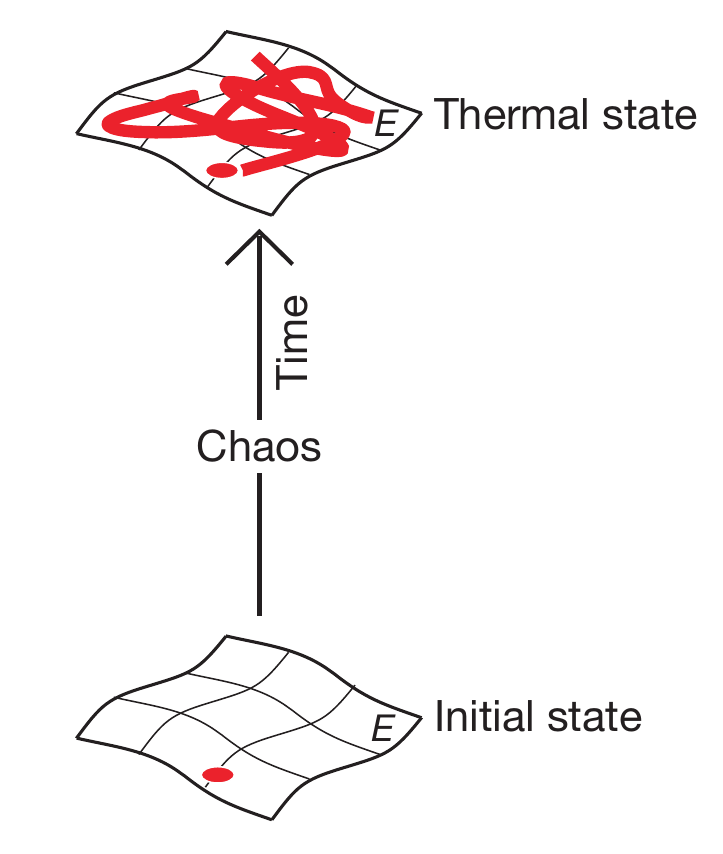[Rigol, Dunjko & Olshanii, Nature 08]

### thermal states are 'typical' states

only regions in phase space contribute to the thermal state, which have a finite probability to be visited (closely) by chaotic trajectories

$\color{darkRed}{\Downarrow}$

regions in phase space which are not sampled by the internal dynamics do not contribute to the thermal state

 caveat for dynamical systems: $\ \$ thermalization at zero temperature? $\ \$ energy not defined?

a given dynamical system may have interesting internal properties,
but in the end what matters is only what can be observed

# textbook: complex systems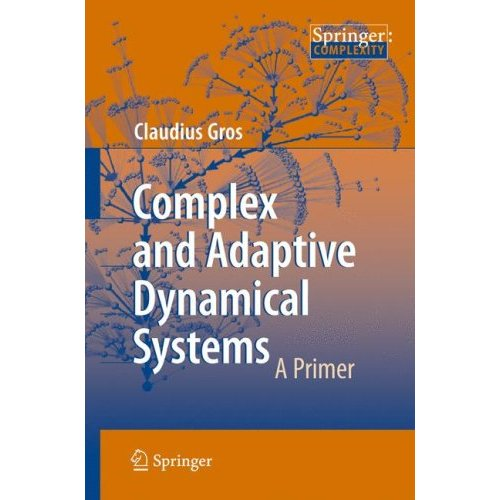• 4-th edition May 2015
• self-organization / pattern formation
• sandpile / branching
• Turing instability / traffic models
• bifurcation theory
• local / global / catastrophes